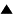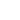# Quasispecies

Quasispecies is a model of informational sequences evolution [1,2]. The evolved population is a set {Sk} of n sequences, k = 1,..., n. Each sequence is a string of N symbols, Ski , i = 1,..., N. The symbols are taken from an alphabet, containing l letters. For example, we can consider a two-letter alphabet (l = 2, Ski = 1, -1 or Ski = G, C) or a four-letter alphabet (l = 4, Ski = G, C, A, U). The sequence length N and the population size n are assumed to be large: N , n >> 1.

Sequences are the model "organisms", they have certain (nonnegative) selective values fk = f(Sk). We assume here, that there is the master sequence Sm , having the maximal selective value. The selective value of any sequence depends only on Hamming distance (the number of different symbols at corresponding places in sequences) between given S and master sequence Sm : f(S) = f(r(S,Sm)) - the smaller is the distance r , the greater is the selective value f . For simplicity we assume here, that values f are not greater than 1.

The evolution process consists of consequent generations. New generation {Sk (t+1)} is obtained from the old one {Sk(t)} by selection and mutations of sequences Sk (t) ; here t is the generation number. The model evolution process can be described formally in the following computer-program-like manner.
 ; Step 0. (Formation of an initial population {Sk (0)} ) For every k = 1 , ..., n, for every i = 1 , ..., N , choose randomly a symbol Ski by setting it to an arbitrary symbol from given alphabet. ; Step 1. (Selection)
 Substep 1.1. (Selection of a particular sequence). Choose randomly a sequence number k*, and select the sequence Sk*(t) (without canceling it from the old population) into the new population {Sk(t+1)} with the probability fk* = f (Sk* (t)). Substep 1.2. (Iteration of the sequences selection, control of the population size). Repeat the substep 1.1 until the number of sequences in the new population reaches the value n .
 Step 2. (Mutations) For every k = 1 , ..., n, for every i = 1 , ..., N , change with the probability P the symbol Ski(t+1) to an arbitrary other symbol of the alphabet. Step 3. (Organization of the iterative evolution). Repeat the steps 1, 2 for t = 0, 1, 2, ...

The evolution character depends strongly on the population size n. If n is very large (n >> lN ), the numbers of all sequences in a population are large and the evolution can be considered as deterministic process. In this case the population dynamics can be described in terms of the ordinary differential equations and analyzed by well known methods. The main result of such an analysis [1-4] are the following conclusions: 1) the evolution process always converges, and 2) the final population is a quasispecie, that is the distribution of the sequences in the neighborhood of the master sequence Sm.

In the opposite case (lN >> n), the evolution process is essentially stochastic, and computer simulations as well as reasonable quantitative estimations can be used to characterize the main evolution features [1,2,5]. At large sequence length N (N > 50) we have just this case for any real population size.

The main evolution features and the estimations in the stochastic case for two-letter alphabet ( l = 2; Ski = 1, -1 ) are described in the child node Estimation of the evolution rate . It is shown that the total number of generations T , needed to converge to a quasispecie at sufficiently large selection intensity, can be estimated by the value
 T ~ (N/2)x(PN)-1, (1)

where P is a mutation intensity. This estimation implies a sufficiently large population size
 n > T, (2)

at which the effect of the neutral selection  can be neglected (see Estimation of the evolution rate, Neutral evolution game for details).

It is interesting to estimate, how effective can be an evolution algorithm of searching. Namely, what is a minimal value of the total number of participants ntotal = nT , which are needed to find a master sequence in evolution process? According to (1) , (2) , to minimize ntotal , we should maximize the mutation intensity P . But at large P , the already found "good" sequences could be lost. "Optimal" mutation intensity P ~ N -1 corresponds approximately to one mutation in any sequence per generation. Consequently, we can conclude that an "optimal" evolution process should involve of the order of
 ntotal = nT ~ N 2 (3)

participants, to find the master sequence.

This value can be compared with the participant number in deterministic and pure random methods of search. The simple deterministic (sequential) method of search (for the considered Hamming-distance-type selective value and two-letter alphabet, Si = 1, -1 ) can be constructed as follows: 1) start with arbitrary sequence S , 2) try to change consequently its symbols: S1 --> - S1 , S2 --> - S2 , ... , by fixing only such symbol changes, those increase the sequence selective value. The total number of sequences, which should be tested in order to find the master sequence Sm in such a manner, is equal to N : ntotal = N . In a pure random search, to find Sm , we need to inspect of the order of 2N sequences : ntotal ~ 2N .

So, we have the following estimations:

 Deterministic search ntotal = N Evolutionary search ntotal ~ N 2 Random search ntotal ~ 2N

Thus, for simple assumptions (Hamming-distance-type selective value and two-letter alphabet), the evolution method of search is essentially more effective than the random one, but it is something worse as compared with the deterministic search.

The Hamming-distance-type model implies that there is unique maximum of the selective value. This is a strong restriction. Using the spin-glass concept (see Spin-glass model of evolution), it is possible to construct a similar model of informational sequences evolution for the case of very large number of the local maxima of a selective value. The evolution rate, restriction on population size, and total number of evolution participants in that model can be also roughly estimated by formulas (1) - (3). But unlike the Hamming-distance model, the spin-glass-type evolution converges to one of the local selective value maxima, which depends on a particular evolution realization.

Conclusion. Quasispecies describes quantitatively a simple information sequence evolution in terms of sequence length, population size, and mutation and selection intensities. This model can be used to characterize roughly the hypothetical prebiotic polynucleotide sequence evolution and to illustrate mathematically general features of biological evolution.

References:

1. M.Eigen. Naturwissenshaften. 1971. Vol.58. P. 465.

2. M.Eigen, P.Schuster. "The hypercycle: A principle of natural self-organization". Springer Verlag: Berlin etc. 1979.

3. C.J.Tompson, J.L.McBride. Math. Biosci. 1974. Vol.21. P.127.

4. B.L.Jones, R.H.Enns, S.S. Kangnekar. Bull. Math. Biol. 1976. Vol.38. N.1. P.15.

5. V.G.Red'ko. Biofizika. 1986. Vol. 31. N.3. P. 511. V.G.Red'ko. Biofizika. 1990. Vol. 35. N.5. P. 831 (In Russian).

6. M. Kimura. "The neutral theory of molecular evolution". Cambridge Un-ty Press. 1983.

 HomeMetasystem Transition TheoryEvolutionary TheoryMathematical Modeling of EvolutionModels of molecular-genetic systems origin Up Prev.Next Down Neutral evolution game Spin-glass model of evolution